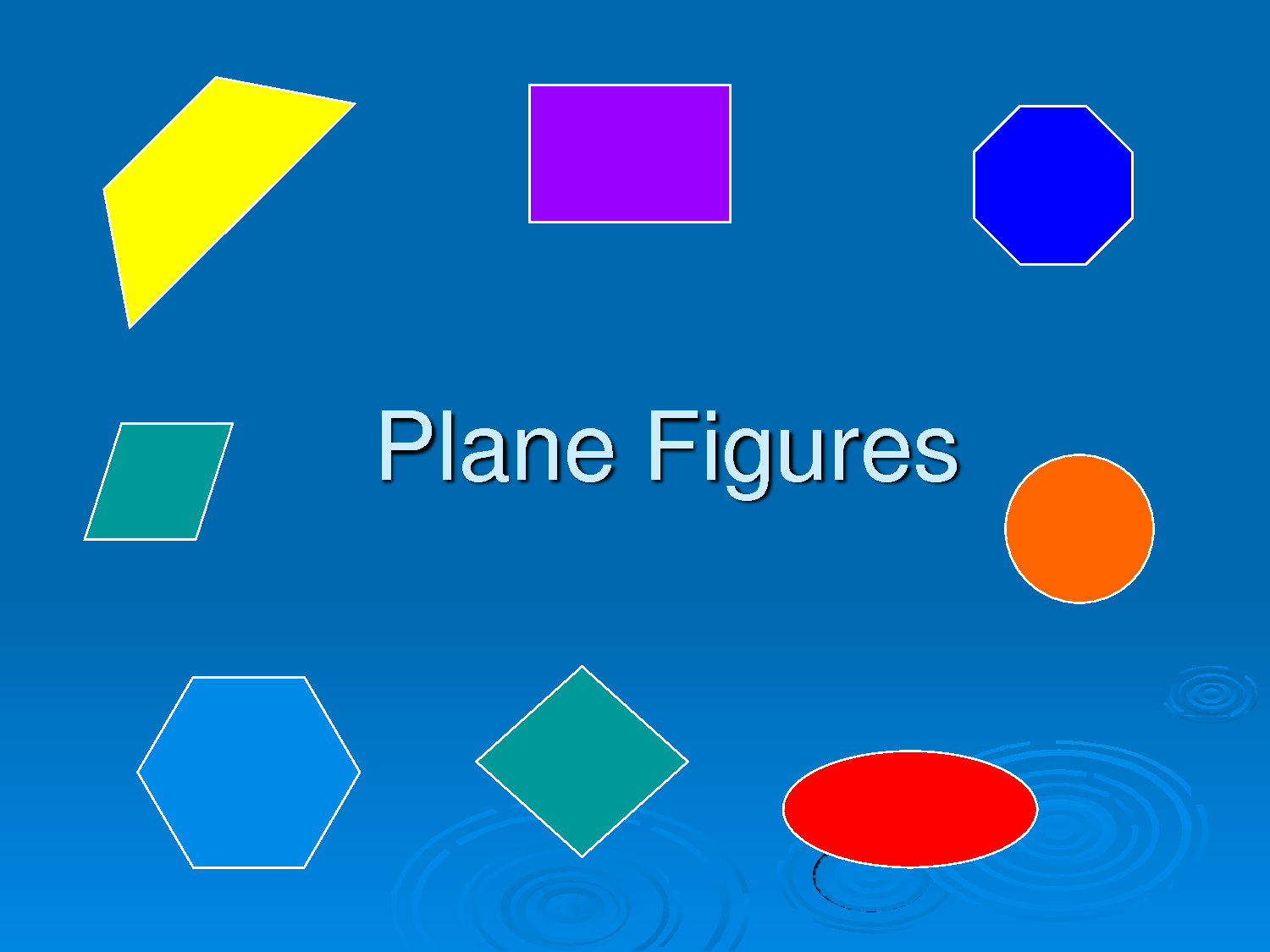# GEOMETRY PLANE SHAPES CONTINUES

Welcome to class!

In today’s class, we will be talking more about geometry plane shapes. Enjoy the class!

### GEOMETRY PLANE SHAPESThe perimeter of a regular polygon, square, rectangle, triangle, trapezium, parallelogram, and circle Area of plain shapes such as square, rectangle, triangle, trapezium, parallelogram, and circle

The different geometrical shapes formula of area and perimeter with examples are discussed below:

#### Perimeter and Area of Rectangle:

● The perimeter of rectangle = 2(l + b).

• Area of rectangle = l × b; (l and b are the length and breadth of the rectangle)
• Diagonal of rectangle = √ (l² + b²)

#### Perimeter and Area of the Square:

• The perimeter of square = 4 × S.
• Area of square = S × S.
• Diagonal of square = S√2; (S is the side of the square)

#### Perimeter and Area of the Triangle:

• Perimeter of triangle = (a + b + c);(a, b, c is 3 sides of a triangle)
• Area of triangle = √ (s (s – a) (s – b) (s – c)); where s is the semi-perimeter of triangle
• S = 1/2 (a + b + c)
• Area of triangle = 1/2 × b × h;(b base, h height)
• Area of an equilateral triangle = (a²√3)/4; (a is the side of the triangle)

#### Perimeter and Area of the Parallelogram:

• The perimeter of parallelogram = 2 (sum of adjacent sides)
• Area of parallelogram = base × height

#### Perimeter and Area of the Rhombus:

• Area of rhombus = base × height
• Area of rhombus = 1/2 × length of one diagonal × length of other diagonal
• The perimeter of rhombus = 4 × side
##### Perimeter and Area of the Trapezium:
• Area of trapezium = 1/2 (sum of parallel sides) × (perpendicular distance between them)
= 1/2 (p₁ + p₂) × h (p₁, p₂ are 2 parallel sides)

#### Circumference and Area of Circle:

• Circumference of circle = 2πr
= πd
Where, π = 3.14 or π = 22/7
r is the radius of the circle
d is the diameter of the circle
● Area of circle = πr²
● Area of ring = Area of the outer circle – Area of the inner circle.

In our next class, we will be talking about Three Dimensional Shapes.  We hope you enjoyed the class.

Should you have any further question, feel free to ask in the comment section below and trust us to respond as soon as possible.

How Can We Make ClassNotesNG Better? - CLICK to Tell Us Now!

1.